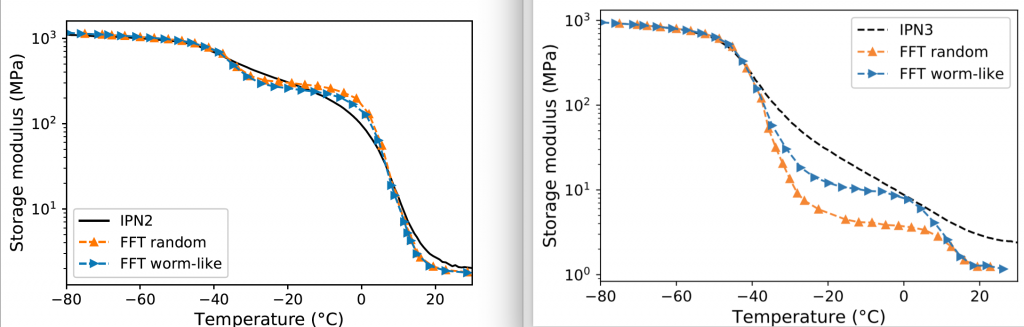# Linear viscoelasticity of IPN – FFT analysis

The objective of the study was to characterize and model the linear viscoelasticity of interpenetrated polymer networks showing two clear distinct phase transitions.
For this purpose we made in lab some acrylate IPNs by UV curing. In order to avoid fracture of the sample during the making process, the first single network is a lightly crosslinked very loose network. The simple networks as well as the IPNs linear viscoelastic properties have been characterized by dynamic mechanical analysis in torsion using solid slender rectangular samples. See post for more details on how to run good torsion DMA tests for solid polymer specimens.
It was found that the acrylate amorphous IPN satisfy to the time-temperature superposition principle and that its linear viscoelastic properties may be well reproduced by a classic generalized Maxwell model.

More interestingly the characterization of the linear viscoelastic properties by temperature sweeps allowed testing some micromechanical modeling in order to better understand the simple network interactions when constrained in the IPN microstructure. Two types of microstructures have been tested, the matrix/inclusion one (based on the Eshelby’s inclusion problem), which does not seem very realistic for IPNs but was the only representation used in the literature so far for multi-scale modeling of such materials, and a cubic lattice representation. The latter one allowed to build a polymer simple network by a classic self-avoiding random walk. Therefore the first single network is assumed as a continuous cube in which the second network is built by a self-avoiding random walk (see Figure below).Lattice microstructure representation of the IPN where the first single network is a continuous homogeneous cube (white voxel) where the second network has been built in by self-avoiding random walk (black voxel).

The use of the FFT method to solve the micromechanics equations is particularly appropriate for lattice microstructures with linear constitutive phases. In order to calculate the dynamic shear modulus of the IPN based on the dynamic shear moduli of the single networks, we have written a FFT python code within the linear viscoelasticity framework. This code as well as the python code to generate the lattice microstructures of interest are now available in the data sharing category.
The satisfactory comparisons between the experimental data of two IPNs, differing in their volume ratios of single networks, and their theoretical behaviors provided by the lattice representation, prove the relevance of the proposed representations. The mechanical interactions of both single networks are defined by this representation.Storage modulus with respect to temperature of IPN2 and IPN3 compared to the modeling estimate – Representation of interest here is blue.

Complete paper including useful references may be found here: https://doi.org/10.1039/d1sm00808k.